# Converting Fractions to Decimals

Rate Lesson 0 stars
Lesson size:
Message preview:
Someone you know has shared lesson with you:

To play this lesson, click on the link below:

https://www.turtlediary.com/lesson/converting-fractions-to-decimals.html

Hope you have a good experience with this site and recommend to your friends too.

Login to rate activities and track progress.
Login to rate activities and track progress.

To convert fractions to decimals, it is important to review what type of numbers fractions and decimals are.

Both are ways to show numbers as parts of a whole.

Fractions are a vertical way to show parts of a whole.

A good way to think of a fraction is like a brownie that has been cut into parts. If there is a pan of brownies cut into 10 equal parts and 4 have been eaten (10 - 4 = 6), then there are 6 brownies left. We would say six out of ten parts remain.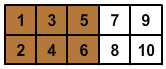The fraction to represent how many brownies are left is:The top number is the number of pieces. It is called the numerator. The bottom number is the total number of pieces. It is called the denominator.

With decimals, the parts are always broken into tenths, hundredths, thousandths, and so on. The digits to the left of the decimal point represent a whole number. The digits to the right of the decimal point represent the parts of a whole.

When we use decimals, we show all the digits horizontally or in a line.As a decimal, the same pan of brownies with six out of ten pieces would be 0.6 or six tenths.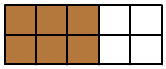And, we just converted the fraction
6
10
to the decimal 0.6.

6
10
= six tenths = 0.6

Notice that before decimal point, there is the digit zero. That is because it more clearly shows, for the decimal number that there is not a whole number: there is only some part of a whole.

We can also add whole numbers to both fractions and decimals to show a number that represents a whole number with some additional parts of a whole.

For example, one whole pan of brownies would be the same as the digit 1 to the left of the decimal point. Since there are no parts of a whole brownie, there would be a zero to the right of the decimal point. One whole is the same as 1 or 1.0.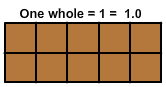A second pan of brownies that had been cut into equal parts, but some pieces had been eaten, is like a fraction or decimal with digits to the right of the decimal point.

If 4 pieces out of 10 had been eaten (and since 10 - 4 = 6), then we would say 0.6 or 6 tenths of the brownie remains. Just count the tenths!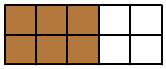If we want to write the whole brownie and the 6 pieces of brownie as a decimal or a fraction, we would write 1.6 or one and six tenths or 1
6
10
.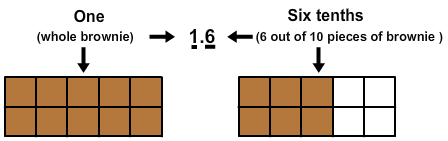Everything is not always cut into 10 equal parts like the brownie. What if something was cut into one hundred parts?

Well, then for fraction the denominator would be 100. And, for decimal we would move two digits to the right of the decimal point.

So, if we had a really large pan of brownies cut into 100 parts and 18 pieces were eaten (100 - 18 = 82), then 0.82 or eighty-two hundredths are the parts of the whole brownie that remain.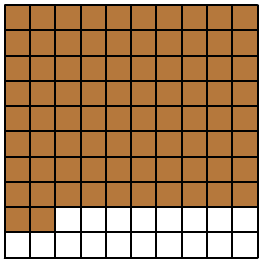82
100
= Eighty-two hundredths = 0.82

Here's a strategy which will help you in converting fractions to decimals:

• When a fraction has a denominator of a tenth, hundredth, thousandth, etc. which matches the place value of decimals, simply place the digits in the numerator to the right of the decimal point.
• To convert a fraction that does not have a denominator of a tenth, hundredth, thousandths, etc. divide the numerator by the denominator. When using long division, if the divisor is larger than the dividend, always add zeros as place holders and round the quotient to the nearest hundredth.

Let's take a look at some examples.

## Example 1

Write
5
10
as a decimal.

Place the numerator 5 to the right of the decimal point to show it has the place value of a tenth.

So,
5
10
= 0.5

## Example 2

Write
43
100
as a decimal.

Place the numerator 43 to the right of the decimal point to show it has a place value of a hundredth.

So,
43
100
= 0.43

## Example 3

Write
224
1000
as a decimal.

Place the numerator 224 to the right of the decimal point to show it has a place value of a thousandth.

So,
224
1000
= 0.224

## Example 4

Write
3
4
as a decimal.

Divide 4 into 3 using long division. Or, type into your calculator 3 ÷ 4.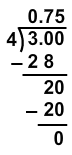So,
3
4
= 0.75

## Example 5

Write
5
8
as a decimal.

Divide 8 into 5 using long division. Or, type into your calculator 5 ÷ 8.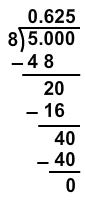So,
5
8
= 0.625

## Example 6

Write
1
3
as a decimal.

Divide 3 into 1 using long division. Or, type into your calculator 1 ÷ 3 .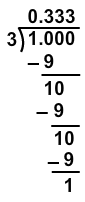In this case, the 3 in the quotient will continue on and on and on. This type of quotient is called a repeating decimal. So, we can just write the answer to the nearest hundredth with a line above the 3 to indicate it is a repeating decimal.

So,
1
3
= 0.33
Summary
• Decimals and fractions both are ways of showing parts of a whole.
• A fraction can be converted to a decimal.
• Strategy to convert fractions to decimals:
• When a fraction has a denominator of a tenth, a hundredth, a thousandth, etc. which matches the place value of decimals, simply place the digits in the numerator to the right of the decimal point. Example:
32
100
= 0.32
• To convert a fraction that does not have a denominator of a tenth, a hundredth, a thousandths, etc. divide the numerator by the denominator. Example: to convert
3
5
to decimal, use the expression 3 ÷ 5 to get the decimal 0.6.
• When using long division, if the divisor is larger than the dividend, always add zeros as place holders and round the quotient to the nearest hundredth.

## More Decimals Games

Become premium member to get unlimited access.